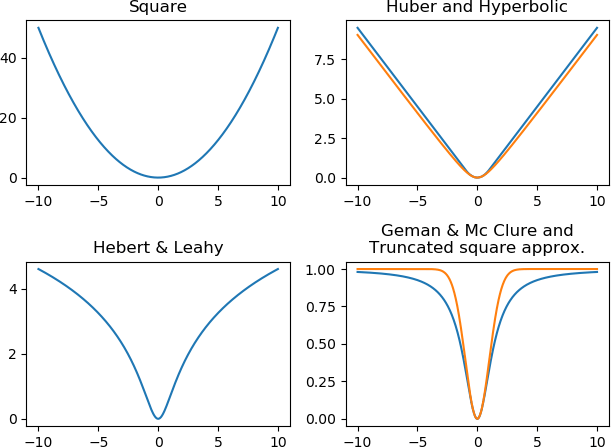# Background¶

Q-MM is Python toolbox to optimise objective functions like

$J(x) = \sum_k \mu_k \Psi_k(V_k x - \omega_k)$

where $$x$$ is the unknown of size $$N$$, $$V_k$$ a matrix or linear operator of size $$M_k \times N$$, $$\omega_k$$ a data fixed vector of size $$M_k$$, $$\mu_k$$ a scalar hyperparameter, and $$\Psi_k(u) = \sum_i \varphi_k(u_i)$$. Q-MM suppose that the scalar functions $$\varphi$$ are differentiable, even, coercive, $$\varphi(\sqrt{\cdot})$$ concave, and $$0 < \dot{\varphi}(u) / u < +\infty$$.

The optimization is done thanks to quadratic surrogate function. In particular, no linesearch is necessary and close form formula for the step are used with guaranteed convergence. The explicit step formula allows fast convergence of the algorithm to a minimizer of the objective function without tuning parameters. On the contrary, the objective must meet the conditions above.

The losses implemented in the toolbox, in addition to the square function, are illustrated below. The Geman & Mc Clure and the truncated square approximation are not coercive.# Example¶

A classical example is the resolution of an inverse problem with the minimization of

$J(x) = \|y - H x\|_2^2 + \mu \Psi(V x)$

where $$H$$ is a low-pass forward model, $$V$$ a regularization operator that approximate object gradient (kind of high-pass filter) and $$\Psi$$ an edge preserving function like Huber. The above objective is obtained with $$k \in \{1, 2\}$$, $$\Psi_1(\cdot) = \|\cdot\|_2^2$$, $$V_1 = H$$, $$\omega_1 = y$$, and $$\omega_2 = 0$$.